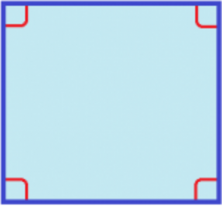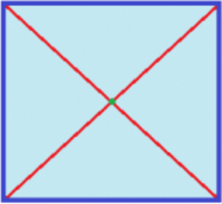Smartick is a fun way to learn math!Jun15

# Geometric Shape: Properties of a Square

Today, we’re going to take a look at a shape that you definitely know already, but maybe you aren’t familiar with all of its main characteristics. I’m talking about the square.

### Geometric Shape: Square

The square is a geometric shape that belongs to the quadrilateral family because it has 4 sides.The 4 sides are the same length and are parallel to each other. What does this exactly mean? It means that 2 sides are parallel to each other, and the other 2 are parallel to each other as well.The 4 interior angles of a square are 90º, or in other words, they’re right angles. When all the angles are added together, they add up to 360º.The two diagonals that a square has are equal in length and the point where they cross is the square’s center.You already knew the square, right? But, did you know about its main characteristics? I hope this post helped you understand!